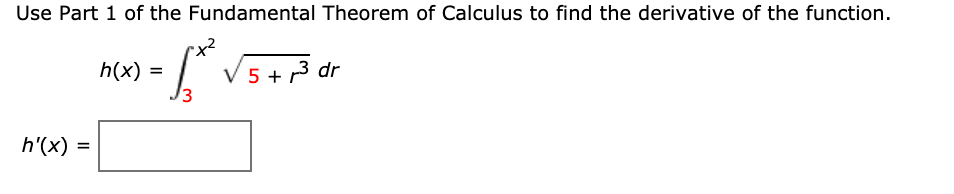# Use Part 1 of the Fundamental Theorem of Calculus to find the derivative of the function. V 5 + 3 dr 13 h(x) = h'(x) =

Questionhelp_outlineImage TranscriptioncloseUse Part 1 of the Fundamental Theorem of Calculus to find the derivative of the function. V 5 + 3 dr 13 h(x) = h'(x) = fullscreen

### Want to see this answer and more?

Experts are waiting 24/7 to provide step-by-step solutions in as fast as 30 minutes!*

*Response times vary by subject and question complexity. Median response time is 34 minutes and may be longer for new subjects.
Tagged in
MathCalculus

### Derivative